## Rounding Numbers in Tableau

by Nick Jastrzebski

Rounding numbers in Tableau is a strange one. If you want to round a decimal to a certain number of places, it’s quite straightforward. The ROUND function lets to specify which field to round and to how many decimals. Rounding to the nearest whole number is simply a 0.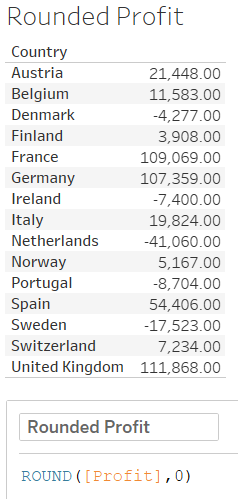Rounding to the nearest whole number.

However, if you want to round to the nearest ten, or nearest hundred, the syntax gets a bit confusing. For nearest ten, you need to round to -1 and nearest 100 is -2. This seems a little odd, but I’ve found a way to make it make some sense, at least to me.

I think of it as where the “rounding barrier” is. To the left of the barrier, there are numbers, to the right of the barrier is only zeros. For ROUND 0, this means the barrier is at the decimal point.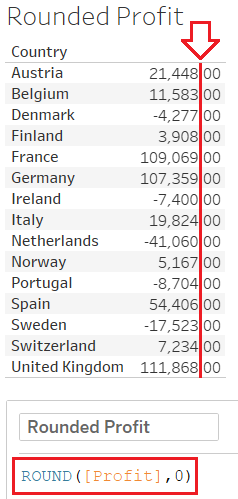The “Rounding Barrier” in red sits at the decimal point when using ROUND 0.

From there, positive rounding moves to the right adding more decimal places to the number’s precision.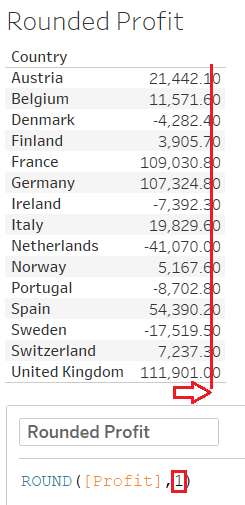The “Rounding Barrier” moves one place to the right for ROUND 1.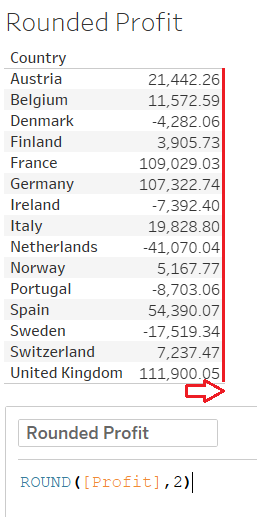ROUND 2 moves the line another digit to the right.

Conversely, negative rounding moves the barrier to the left. So for -1, the rounding barrier moves one digit to the left of the decimal, rounding all the numbers to the nearest 10!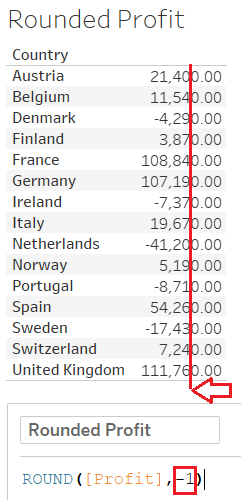ROUND -1 moves the “Rounding Barrier” to the left, decreasing precision.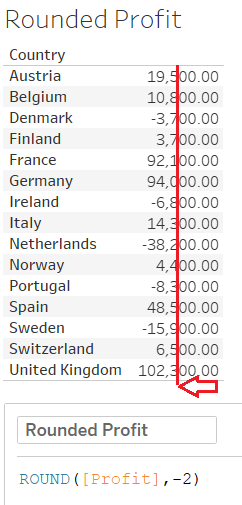ROUND -2 gives values to the nearest 100, with the “Rounding Barrier” two places to the left of the decimal.

Hopefully this makes the syntax for rounding in Tableau a little easier to understand. I know it caused me to scratch my head once or twice!Nick Jastrzebski

Fri 30 Nov 2018

Fri 02 Nov 2018

Thu 01 Nov 2018